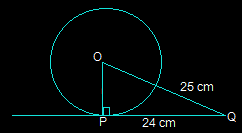Guru

# From a point Q, the length of the tangent to a circle is 24 cm and the distance of Q from the centre is 25 cm. The radius of the circle is (A) 7 cm (B) 12 cm (C) 15 cm (D) 24.5 cm Q.1

• 0

How i solve the problem from class 10th ncert of exercise 10.2 of circles chapter of question no.1, i think this an important question for class 10th, give me the best way to solve this problem From a point Q, the length of the tangent to a circle is 24 cm and the distance of Q from the centre is 25 cm. The radius of the circle is (A) 7 cm (B) 12 cm (C) 15 cm (D) 24.5 cm

Share

1. First, draw a perpendicular from the center O of the triangle to a point P on the circle which is touching the tangent. This line will be perpendicular to the tangent of the circle.So, OP is perpendicular to PQ i.e. OP ⊥ PQ

From the above figure, it is also seen that △OPQ is a right angled triangle.

It is given that

OQ = 25 cm and PQ = 24 cm

By using Pythagoras theorem in △OPQ,

OQ2 = OP2 +PQ2

(25)2 = OP2+(24)2

OP2 = 625-576

OP2 = 49

OP = 7 cm

So, option A i.e. 7 cm is the radius of the given circle.

• 0